# Pressure loss calculator, head loss in pipe

## Characteristics of the pipeline

Diameter: mm

The length of pipe: m

Flow: l/min

Fluid (temperature 20° C):
Water
Benzine
Petroleum
Kerosene
Hydraulic oil

Piping Material:
Steel
Cast iron
Copper
Aluminum
Rubber
Concrete
Polypropylene

## Pressure drop calculation algorithm

Checking calculation results.

Calculate values:
• Mean velocity of the fluid
•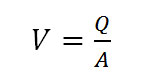Q - volumetric flow rate, m3/s, A - pipe's cross-sectional area, m2, A=πd2/4, d - pipe bore, m
• Reynolds number Re
•V - mean velocity of the fluid, m/s, d - diameter, m, ν - kinematic viscosity, m2/c, Rr - hydraulic radius, for round pipe R=d/4, d - diameter, m

Darcy friction factor formula may be applicable depends upon the type of flow that exists.

• The Darcy friction factor for laminar flow Re<2000 is given by the following formula:
•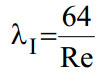• Which friction factor for transition between laminar and turbulent flow 2000<Re<4000 - is given by the following formula:
•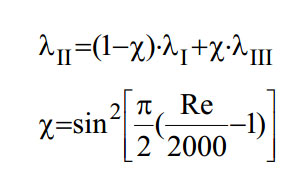• The Darcy friction factor for turbulent flow Re>4000 is given by the following formula.
• к=Δ/d, Δ - absolute roughness coefficient.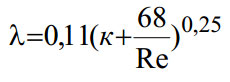### The head loss calculation

The head loss in a pipe, tube or duct can be calculated with the Darcy-Weisbach equation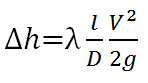### The pressure drop calculation

The pressure drop can be calculated with the Darcy-Weisbach equation.ρ - density of the liquid kg/m3, g - acceleration of gravity

Languages
Spanish (ES) Deutsch (DE)
Featured articles
How to read hydraulic circuits
Hydraulic cylinders
Hydraulic accumulators
Best calculators
Pressure loss calculator
Calculating the system curve
Hydraulic cylinder force calculator
My Youtube channel
This site uses cookies to store information on your computer. Details